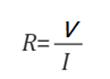# Raspberry Pi_Eng_25.3.1 Current, Resistance, and Voltage

#### Published Book on Amazon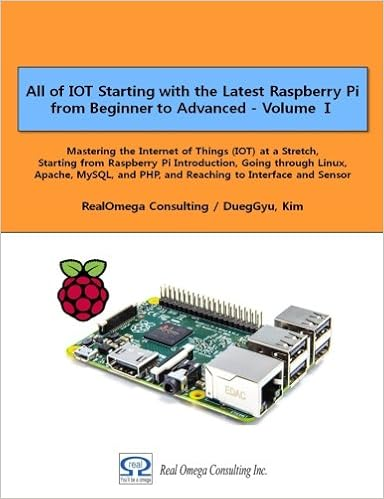All of IOT Starting with the Latest Raspberry Pi from Beginner to Advanced – Volume 1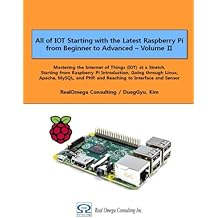All of IOT Starting with the Latest Raspberry Pi from Beginner to Advanced – Volume 2

#### 출판된 한글판 도서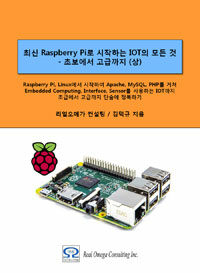최신 라즈베리파이(Raspberry Pi)로 시작하는 사물인터넷(IOT)의 모든 것 – 초보에서 고급까지 (상)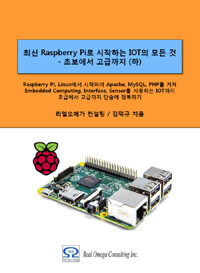최신 라즈베리파이(Raspberry Pi)로 시작하는 사물인터넷(IOT)의 모든 것 – 초보에서 고급까지 (하)

## 25.3Basics of Electricity/Electronics

Here, we will explain the basics of electricity and electronics.

### 25.3.1Current, Resistance, and Voltage

#### 25.3.1.1Current

There are many free electrons in the conductor. When a voltage is applied to a conductor, the free electrons are attracted to the anode (+ pole), which is the current. Although the electrons flow from the cathode (- pole) to the anode ((+pole), the current is said to flow from the anode to the cathode as opposed to the direction of the electrons, because it has been decided to use what has been being used from the past.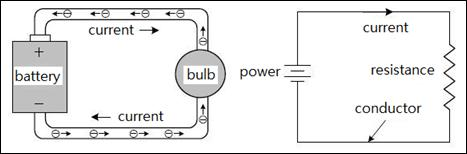Figure 25‑4 Current

"electron" has the nature of (-) electricity, which is called "electric charge". The quantity of electric charge that one electron has is defined as 1.6×10^-19 Coulombs. Therefore, 1C (Coulomb) is the electric charge that 1/(1.6 x 10^-19) = 6.25 x 10^18 electrons have.

The flow of current through a conductor is similar to the flow of water through a water pipe. In a water pipe of a certain thickness, when the water pressure is high, a large amount of water flow and when the water pressure is low, a small amount of water flow. Similarly, in the case of current in a wire of a certain thickness, much amount of current flow when the voltage is high, and a small amount of current flow when the voltage is low.

The magnitude of current is denoted by A (Ampere), where 1A means the transfer of 6.25×10^18 electrons, ie, the electric charge of 1 Coulomb, per second.

#### 25.3.1.2Voltage and Electric Potential

Voltage is the amount of force that pushes electrons to move, like a battery. Therefore, the voltage is the same concept as the water pressure in the water pipe. The unit of voltage is expressed in V (Volt). 1V is the magnitude of the voltage that can flow current of 1A through 1Ω resistor.

There is a term electric potential which is used analogous to voltage. Electric potential refers to the potential energy that a unit of electric charge has at a specific point in an electric field. The magnitude of potential energy is usually measured based on ground (0V).

Voltage, on the other hand, refers to the difference in electric potential energy between two points, which compares relatively how many charged particles are at two points. In other words, a voltage means a difference in potential energy of a unit of electric charge, that is, a electric potential difference. Current flows by this voltage.

In the figure below, the relationship between potential and voltage is shown with the reference potential set at 0V. Between the potential 1.5V and the potential 3V, the potential difference is 1.5V, which means that the voltage is 1.5V.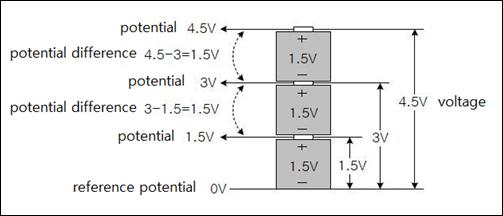Figure 25‑5 Voltage and potential

#### 25.3.1.3Resistance

Resistance is a property that prevents current from flowing. Every object has its own electrical resistance. A substance with a low electrical resistance is called a conductor, and an object with a very high resistance is called a nonconductor or insulator. The electrical resistance is similar to the way in which rust and scrubbing in a water pipe interferes with the flow of water.

The resistance is expressed in Ω (ohm) unit. Specific resistance (or resistivity) refers to the inherent resistance characteristic of a material, expressed in Ω•m.Figure 25‑6 Resistance

1Ω•m refers to the resistance value obtained by measuring the resistance at both ends of a conductor plate placed on both sides of a cube of 1m length and height as shown in the above figure. If the length of each side is 1cm, this is 1Ω•cm. The Specific resistances of some materials are as follows

 material ( insulator ) Specific resistance ρ [Ω·m] Copper 1.72×10^−8 aluminum 2.75×10^−8 iron 9.8×10^−8 Glass 10^9 ~ 10^11 Ceramic 3×10^11 Bakelite 10^6 ~ 10^10

The magnitude of the resistance is calculated by the following equation. The resistance of a material is proportional to its length and inversely proportional to its cross-sectional area.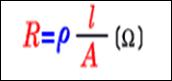R         --resistance

ρ          -- specific resistance

l           -- length

A         -- area

#### 25.3.1.4Ohm's Law

Ohm in Germany discovered the law of the relationship between voltage, current and resistance, which is called Ohm's law. This rule is that the magnitude of the current flowing through the circuit is proportional to the voltage applied to the circuit and is inversely proportional to the resistance of the circuit. In other words, the current flowing in the circuit is expressed by the following equation.We can transform Ohm's law as follows, which means that multiplying the current flowing in a circuit by the resistance of that circuit is the voltage applied to that circuit.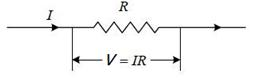V = IR

The above equation can also be transformed as follows, which means that dividing the voltage applied to the circuit by the current flowing through the circuit becomes the resistance of the circuit.